# Numeration System

Also found in: Dictionary, Thesaurus.

## numeration system

[‚nü·mə′rā·shən ‚sis·təm]
(mathematics)
An orderly method of representing numbers by numerals in which each numeral is associated with a unique number. Also known as number system; numeral system.

## Numeration System

(or numeral system), a set of methods for naming and representing numbers.

The most advanced numeration systems are based on the positional, or place-value, principle. According to this principle, the value of a numerical symbol, or numeral, in the representation of a number depends on the position of the symbol in the representation. In such systems, a certain number n of units in a given place are represented by a single unit in the next higher place, n units in that place are represented by a single unit in the next higher place, and so on. The number n is called the base, or radix, of the system.

An example of a positional system is the modern decimal number system, whose base n = 10. The first ten numbers are represented in the decimal system by the numerals 0, 1,..., 9 (seeDECIMAL SYSTEM). Despite the seeming naturalness of this system, it is the result of a long historical development. The origin of the decimal system can be traced to the practice of counting on fingers.

Systems with other bases have also been used. Examples of bases that have been employed are five, 12 (counting by dozens), 20, 40, and 60. Traces of the use of the base 20 remain in French: 80, for example, is quatre-vingts, which literally means “four twenties.” The binary system, for which n = 2, is often used in scientific research and in computation by means of computers (seeBINARY SYSTEM OF NUMERATION).

Primitive peoples lacked well-developed numeration systems. As recently as the 19th century, many tribes in Australia and Polynesia had only two numerals: a numeral representing “one” and a numeral representing “two.” Combinations of these numerals yielded the numbers three (two-one), four (two-two), five (two-two-one), and six (two-two-two). Numbers greater than six were not individualized and were referred to as many.

As social and economic life developed, it became necessary to create systems that could be used to count and represent increasingly larger collections of objects. One of the earliest numeration systems was the Egyptian hieroglyphic system, which was developed between 3000 and 2500 B.C. A decimal, nonpositional system, it used only the additive principle to express numbers: the numbers represented by a group of numerals standing together were added. Symbols existed for one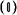, ten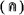, 100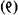, and other powers of ten up to 107. The number 343 was written Written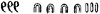. Examples of similar systems are the Greek He-rodianic, the Roman (seeROMAN NUMERALS), and the Syrian.

Alphabetic systems, such as the Ionian, Slavic (seeSLAVIC NUMERALS), Hebrew, Arabic, Georgian, and Armenian, are more sophistocated than the above types. The Ionian system, which developed in the Greek colonies in Asia Minor in the mid-5th century B.C., was apparently the first alphabetic system. In alphabetic numeration systems, the numbers from one to nine and all tens and hundreds were usually denoted by consecutive letters of the alphabet; lines were sometimes placed above the letters in order to distinguish numbers from words. In the Ionian system, the number 343 was written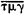; here,denotes 300,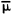40, and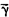3. In the Slavic system, 343 was written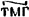. Alphabetic systems permitted numbers to be written far more compactly than in earlier systems. Moreover, arithmetic operations could be performed much more easily on numbers written in alphabetic systems. Arbitrarily large numbers, however, could not be written in such systems. The Greeks expanded the Ionian system by using for the numbers 1,000, 2,000,..., 9,000 the same letters as for 1, 2,..., 9, with a distinguishing stroke being placed to the lower left of the letter. Thus,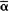denoted 1,000,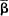denoted 2,000, and so on. The new symbol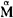was introduced for 10,000. Nevertheless, the Ionian system proved to be unsuitable for the astronomical computations carried out in the Hellenistic period, and the Greek astronomers of that time began combining the alphabetic system with the Babylonian sexagesimal system.

The numeration system of the ancient Babylonians is the earliest known system based on the positional principle. In this system, which was developed around 2000 B.C., all numbers were written by means of the two symbols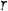(one) and(ten). Numbers up to 60 were expressed by combinations of the two symbols on the basis of the additive principle. The symbol) was again used, in a new position, to express the number 60: the symbol now represented a unit of higher order. The additive principle was again used to write numbers from 60 to 3,600, the symbol for one was again used to express the number 3,600, and so on. In this system, the number 343 = 5·60 + 4·10 + 3 was written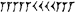. Since, however, the system lacked a symbol for zero, that is, a symbol for indicating a missing position. The notation was not single-valued (seeCUNEIFORM MATHEMATICAL TEXTS).

Another numeration system based on the positional principle was developed in the mid-first millennium A.D. by the Maya Indians, who inhabited the Yucatán Peninsula in Central America. The Maya had two numeration systems: one resembled the Egyptian system and was used in daily life, and the other was a vigesimal positional system used in calendar calculations. The second system had a symbol for zero, and, as in our modern system, the notation was single-valued.

The modern decimal positional system arose on the basis of a system developed in India not later than the fifth century. Until the adoption of this system, the systems used in India were based not only on the additive principle but also on the multiplicative principle, according to which a unit in a given place was multiplied by the number standing to its left. The ancient Chinese system and some other systems were of much the same nature. For example, if the number three is denoted by the symbol III and the number ten is denoted by the symbol X, then 30 would be written IIIX (three tens). Such number systems may have paved the way for the creation of the decimal positional system.

The decimal positional system theoretically permits arbitrarily large numbers to be written. The system has a compact notation and is convenient for performing arithmetic operations. For these reasons, it spread from India to the east and west shortly after its appearance. The system was presented in Arabic manuscripts of the ninth century, reached Spain in the tenth century, and appeared in other European countries in the early 12th century. The new system was called the Arabic system because it became known in Europe through Latin translations from the Arabic. It did not come into wide use in science and daily life until the 16th century. In Russia, the system began spreading in the 17th century and supplanted the alphabetic system at the very beginning of the 18th century. With the introduction of decimal fractions, the decimal positional system became an all-purpose means for expressing the real numbers.

### REFERENCES

Cajori, F. Istoriia elementarnoi matematiki s ukazaniiami na metody prepodavaniia, 2nd ed. Odessa, 1917. (Translated from English.)
Löffler, E. Tsifry i tsifrovye sistemy kul’turnykh narodov v drevnosti i v novoe vremia. Odessa, 1913. (Translated from German.)
Vygodskii, M. Ia. Arifmetika i algebra v drevnem mire, 2nd ed. Moscow, 1967.
Bashmakova, I. G., and A. P. Iushkevich. “Proiskhozhdenie sistem schisleniia.” In Entsiklopediia elementarnoi matematiki, book 1. Moscow-Leningrad, 1951.

I. G. BASHMAKOVA

References in periodicals archive ?
to lay a foundation for mental composition and decomposition of numbers and the later learning of addition facts and the written numeration system.
The Chinese numeration system is a decimal (base-ten) system, unlike other systems such as the Babylonian (sexagesimal or base-sixty) or the Mayan (vigesimal or base-twenty).
Lack of linguistic support may contribute to the challenges young children face in constructing their knowledge of our numeration system in the early grades (Miura et al.
First we looked at the written symbols of the Egyptian numeration system.
Moreover, Chinese teachers believe that students should use 10 as a bridge because of its importance in the base-ten numeration system.
The Hindu-Arabic numeration system is considered one of the most important inventions in the history of humankind.
When reading the book in one sitting, one gets a sense of the time that elapsed from the beginning of recording numbers to the development of the numeration system we use today.
The Hindu-Arabic numeration system is based on four basic properties that constitute place value:
Fuson and Briars (1990) report that using base-ten blocks to solve a wide variety of problems helped the second graders in their research study begin to develop the concepts of multiunits that are essential to understanding our numeration system.
Sama was the lead engineer in the design and implementation of the controls and interface for the Fan Assessment Numeration Systems (FANS) project.
numerals: the Hindu-Arabic numerals (0, 1, 2, 3, 4, 5, 6, 7, 8, and 9) and also those of other numeration systems such as Roman (I, V, X, D, C, L, and M) that are used, in various combinations, to represent numbers of all types;
The discussions included the history of various numeration systems, telling time in connection to the numerals and by the direction of the sun, and learning about how the sun was once widely used to keep time.

Site: Follow: Share:
Open / Close